# Coordinates of square vertices

I have coordinates of square vertices A / -3; 1/and B/1; 4 /. Find coordinates of vertices C and D, C 'and D'. Thanks Peter.

Result

x2 =  2.97
y2 =  -0.596
x3 =  -1.03
y3 =  -3.596
x4 =  -0.97
y4 =  8.596
x5 =  -4.97
y5 =  5.596

#### Solution:

$x_{ 0 } = -3 \ \\ y_{ 0 } = 1 \ \\ \ \\ x_{ 1 } = 1 \ \\ y_{ 1 } = 4 \ \\ \ \\ a = \sqrt{ (x_{ 0 }-x_{ 1 })^2+(y_{ 0 }-y_{ 1 })^2 } = \sqrt{ ((-3)-1)^2+(1-4)^2 } = 5 \ \\ \ \\ \tan α = \dfrac{ y_{ 0 }-y_{ 1 } }{ x_{ 0 }-y_{ 1 } } = \dfrac{ 1-4 }{ (-3)-4 } = \dfrac{ 3 }{ 7 } \doteq 0.4286 \ \\ \ \\ α = \arctan (\dfrac{ y_{ 0 }-y_{ 1 } }{ x_{ 0 }-y_{ 1 } } ) = \arctan (\dfrac{ 1-4 }{ (-3)-4 } ) \doteq 0.4049 \ rad \ \\ \ \\ dx = a \cdot \ \cos(α) = 5 \cdot \ \cos(0.4049) \doteq 4.5957 \ \\ dy = a \cdot \ \sin(α) = 5 \cdot \ \sin(0.4049) \doteq 1.9696 \ \\ \ \\ x_{ 2 } = x_{ 1 } + dy = 1 + 1.9696 \doteq 2.9696 = 2.97$
$y_{2} =y_{ 2 } = y_{ 1 } - dx = 4 - 4.5957 \doteq -0.5957 = -0.596$
$x_{ 3 } = x_{ 0 } + dy = (-3) + 1.9696 \doteq -1.0304 = -1.03$
$y_{ 3 } = y_{ 0 } - dx = 1 - 4.5957 \doteq -3.5957 = -3.596$
$x_{4} =x_{ 4 } = x_{ 1 } - dy = 1 - 1.9696 \doteq -0.9696 = -0.97$
$y_{4} =y_{ 4 } = y_{ 1 } + dx = 4 + 4.5957 \doteq 8.5957 = 8.596$
$x_{ 5 } = x_{ 0 } - dy = (-3) - 1.9696 \doteq -4.9696 = -4.97$
$y_{ 5 } = y_{ 0 } + dx = 1 + 4.5957 \doteq 5.5957= 5.596 \ \\ \ \\ a_{ 2 } = \sqrt{ (x_{ 0 }-x_{ 3 })^2+(y_{ 0 }-y_{ 3 })^2 } = \sqrt{ ((-3)-(-1.0304))^2+(1-(-3.5957))^2 } \doteq 5.0004 \ \\ a_{ 3 } = \sqrt{ (x_{ 1 }-x_{ 2 })^2+(y_{ 1 }-y_{ 2 })^2 } = \sqrt{ (1-2.9696)^2+(4-(-0.5957))^2 } \doteq 5.0004 \ \\ a_{ 4 } = \sqrt{ (x_{ 2 }-x_{ 3 })^2+(y_{ 3 }-y_{ 2 })^2 } = \sqrt{ (2.9696-(-1.0304))^2+((-3.5957)-(-0.5957))^2 } = 5 \ \\ \ \\ b_{ 2 } = \sqrt{ (x_{ 0 }-x_{ 5 })^2+(y_{ 0 }-y_{ 5 })^2 } = \sqrt{ ((-3)-(-4.9696))^2+(1-5.5957)^2 } \doteq 5.0002 \ \\ b_{ 3 } = \sqrt{ (x_{ 1 }-x_{ 4 })^2+(y_{ 1 }-y_{ 4 })^2 } = \sqrt{ (1-(-0.9696))^2+(4-8.5957)^2 } \doteq 5.0004 \ \\ b_{ 4 } = \sqrt{ (x_{ 4 }-x_{ 5 })^2+(y_{ 4 }-y_{ 5 })^2 } = \sqrt{ ((-0.9696)-(-4.9696))^2+(8.5957-5.5957)^2 } \doteq 5.0002$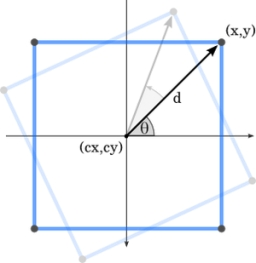Our examples were largely sent or created by pupils and students themselves. Therefore, we would be pleased if you could send us any errors you found, spelling mistakes, or rephasing the example. Thank you!

Leave us a comment of this math problem and its solution (i.e. if it is still somewhat unclear...):Be the first to comment!Tips to related online calculators
For Basic calculations in analytic geometry is helpful line slope calculator. From coordinates of two points in the plane it calculate slope, normal and parametric line equation(s), slope, directional angle, direction vector, the length of segment, intersections the coordinate axes etc.
Pythagorean theorem is the base for the right triangle calculator.
Most natural application of trigonometry and trigonometric functions is a calculation of the triangles. Common and less common calculations of different types of triangles offers our triangle calculator. Word trigonometry comes from Greek and literally means triangle calculation.

## Next similar math problems:

1. Regular n-gonWhich regular polygon have a radius of circumscribed circle r = 10 cm and the radius of inscribed circle p = 9.962 cm?
2. Two forcesTwo forces with magnitudes of 25 and 30 pounds act on an object at angles of 10° and 100° respectively. Find the direction and magnitude of the resultant force. Round to two decimal places in all intermediate steps and in your final answer.
3. Right angled triangle 3Side b = 1.5, hypotenuse angle A = 70 degrees, Angle B = 20 degrees. Find its unknown sides length.
4. Ratio iso triangleThe ratio of the sides of an isosceles triangle is 7:6:7 Find the base angle to the nearest answer correct to 3 significant figure.
5. Trapezoid MOThe rectangular trapezoid ABCD with right angle at point B, |AC| = 12, |CD| = 8, diagonals are perpendicular to each other. Calculate the perimeter and area of ​​the trapezoid.
6. Tetrahedral pyramidDetermine the surface of a regular tetrahedral pyramid when its volume is V = 120 and the angle of the sidewall with the base plane is α = 42° 30´.
7. The aspect ratioThe aspect ratio of the rectangular triangle is 13: 12: 5. Calculate the internal angles of the triangle.
8. One side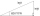One side is 36 long with a 15° incline. What is the height at the end of that side?
9. Church tower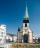Archdeacon church in Usti nad Labem has diverted tower by 186 cm. The tower is 65 m high. Calculate the angle by which the tower is tilted. Result write in degree's minutes.
10. Isosceles triangle 10In an isosceles triangle, the equal sides are 2/3 of the length of the base. Determine the measure of the base angles.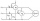Two wattmeters are connected to measuring power in a 3 phase balanced load. Determine the total power and power factor if the two wattmeters read 1000 watts each (1) both positive and (2) second reading is negativeCalculate the diagonal length of the regular pentagon: a) inscribed in a circle of radius 12dm; b) a circumscribed circle with a radius of 12dm.Calculate the length of the remaining two sides and the angles in the rectangular triangle ABC if a = 10 cm, angle alpha = 18°40'.The angle of a straight road is approximately 12 degrees. Determine the percentage of this road.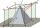The height of the regular quadrangular pyramid is 6 cm, the length of the base is 4 cm. What is the angle between the ABV and BCV planes?Approximately at what height is the cloud we see under an angle of 26°10' and see the Sun at an angle of 29°15' and the shade of the cloud is 92 meters away from us?Between points A and B is 50m. From A we see a tree at an angle 18°. From point B we see the tree in three times bigger angle. How tall is a tree?